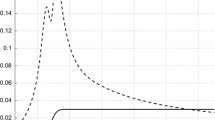# Fourier Inversion Formulas in Option Pricing and Insurance

## Abstract

Several authors have used Fourier inversion to compute prices of puts and calls, some using Parseval’s theorem. The expected value of max (SK, 0) also arises in excess-of-loss or stop-loss insurance, and we show that Fourier methods may be used to compute them. In this paper, we take the idea of using Parseval’s theorem further: (1) formulas requiring weaker assumptions; (2) relationship with classical inversion theorems for probability distributions; (3) formulas for payoffs which occur in insurance. Numerical examples are provided.

This is a preview of subscription content, log in via an institution to check access.

Price excludes VAT (USA)
Tax calculation will be finalised during checkout.

### Similar content being viewed by others### Fourier-Based Valuation Methods in Mathematical Finance### Approximate option pricing and hedging in the CEV model via path-wise comparison of stochastic processes

Vladislav Krasin, Ivan Smirnov & Alexander Melnikov

Moawia Alghalith

## References

• M. Abramowitz, and I. Stegun, Handbook of Mathematical Functions: With Formulas, Graphs and Mathematical Tables, Dover: New York, 1970.

• G. Bakshi, and D. B. Madan, “Spanning and derivative-security valuation,” Journal of Financial Economics vol. 55 pp. 205–238, 2000.

• K. Borovkov, and A. Novikov, “A new approach to calculating expectations for option pricing,” Journal of Applied Probability vol. 39 pp. 889–895, 2002.

• P. Carr, and D. B. Madan, “Option valuation using the fast Fourier transform,” Journal of Computational Finance vol. 2 pp. 61–73, 1999.

• F. Dufresne, and H. U. Gerber, “Risk theory for the compound Poisson process that is perturbed by diffusion,” Insurance: Mathematics and Economics vol. 10 pp. 51–59, 1991.

• H. J. Furrer, “Risk processes perturbed by a α–stable Lévy motion,” Scandinavian Actuarial Journal vol. 1998 pp. 59–74, 1998.

• S. L. Heston, “A closed-form solution for options with stochastic volatility with application to bond and currency options,” Review of Financial Studies vol. 6 pp. 327–343, 1993.

• M. Kendall, and A. Stuart, The Advanced Theory of Statistics, Fourth Edition. Griffin: London, 1977.

• N. N. Lebedev, Special Functions and Their Applications, Dover: New York, 1972.

• R. W. Lee, “Option pricing by transform methods: extensions, unification, and error control,” Journal of Computational Finance vol. 7 pp. 51–86, 2004.

• A. L. Lewis, “A simple option formula for general jump–diffusion and other exponential Lévy processes,” OptionCity.net publications: http://optioncity.net/pubs/ExpLevy.pdf , 2001.

• E. Lukacs, Characteristic Functions, Fourth Edition. Griffin: London, 1970.

• P. Malliavin, Integration and Probability, Springer Verlag: New York, 1995.

• S. Raible, Lévy Processes in Finance: Theory, Numerics, and Empirical Facts. Ph.D. Dissertation, Faculty of Mathematics, University of Freiburg, Germany, 2000.

• G. Samorodnitsky, and M. S. Taqqu, Stable Non-Gaussian Random Processes: Stochastic Models with Infinite Variance, Chapman & Hall: New York, 1994.

• K. Sato, Lévy Processes and Infinitely Divisible Distributions, Cambridge University Press: Cambridge, 1999.

## Author information

Authors

### Corresponding author

Correspondence to Daniel Dufresne.

## Rights and permissions

Reprints and Permissions

Dufresne, D., Garrido, J. & Morales, M. Fourier Inversion Formulas in Option Pricing and Insurance. Methodol Comput Appl Probab 11, 359–383 (2009). https://doi.org/10.1007/s11009-007-9049-z

• Revised:

• Accepted:

• Published:

• Issue Date:

• DOI: https://doi.org/10.1007/s11009-007-9049-z